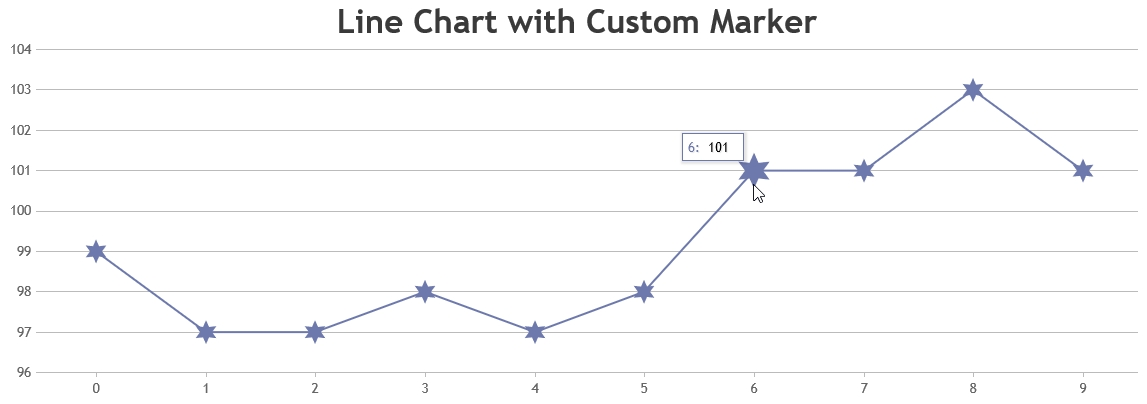#37383

Thanks for being a valuable customer of CanvasJS.

1. Add marker drawing logic in the end of drawMarker method of canvasjs.js (Source-Code) downloaded from My Account page.

``````else if(markerType === "customMarkerType") {
//marker drawing logic
}``````

2. Define the newly added markerType in dataseries

``````data: [{
.
.
markerType: "customMarkerType"
.
}]``````

``````//Add the below lines at the end of drawMarker method
else if (markerType === "star") {
var points = 6;
ctx.strokeStyle = markerColor;

ctx.beginPath();
ctx.moveTo(x, y + markerSize);
for (var i = 0; i < 2 * points + 1; i++) {
var r = (i % 2 == 0) ? markerSize : markerSize / 2;
var a = Math.PI * i / points;
ctx.lineTo(x + r * Math.sin(a), y + r * Math.cos(a));
};
ctx.closePath();

if (markerColor)
ctx.fill();
}

//Define marker-type in chart-options
data: [{
...
markerType: "star",
}]``````Vishwas R
Team CanvasJS